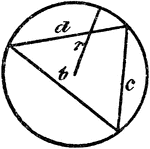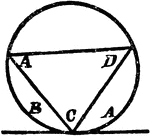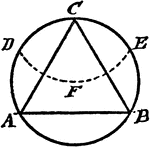### Triangle Inscribed In A Circle

An illustration showing a triangle with sides b, c, and d inscribed in a circle with radius r.### Triangle Inscribed In A Circle

An illustration showing a triangle with angles A, C, and D inscribed in a circle which is tangent to…### Construction Of An Equilateral Triangle Inscribed In A Circle

An illustration showing how to construct an equilateral triangle inscribed in a circle. "With the radius…